# ISEE Upper Level Quantitative : How to find mode

## Example Questions

2 Next →

### Example Question #11 : How To Find Mode

Consider the following set of data whereis an unknown value: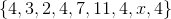Which one is greater: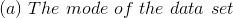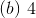It is not possible to compare the mean and the mode based on the information given.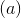is greaterand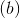are equal.is greaterandare equal.

Explanation:

The mode of a set of data is the value which occurs most frequently. The value ofappears at least four times in the set. So regardless of the value of, none of the other values can appear more than two times. Sois the mode.

### Example Question #12 : How To Find Mode

A data set has six known quantities and one unknown quantity, as follows: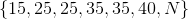Which is the greater quantity?

(A) The mode of the set if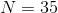(b) The mode of the set if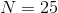(A) is greater

(B) is greater

(A) and (B) are equal

It is impossible to determine which is greater from the information given

(A) is greater

Explanation:

The mode of a data set is the element that occurs most frequently.

If, the set becomes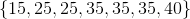.

The mode is 35, which occurs three times, more than any other element.

If, the set becomes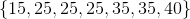.

The mode is 25, which occurs three times, more than any other element.

(A) is greater.

### Example Question #13 : How To Find Mode

Consider the data set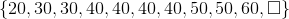.

Which of the following elements replaces the box to make the data set bimodal?

(A)(B)(C)(C) only

(B) only

(A), (B), and (C) are all correct.

None of (A), (B), or (C) are correct.

(A) only

None of (A), (B), or (C) are correct.

Explanation:

At current, 40 appears four times, more than any other value. For a set to be bimodal, it needs to have two modes; that is, another value would have to appear four times as well. Regardless of whether 30, 50, 60, or any other value replaces the box, this is impossible. The set cannot be made bimodal by adding one element.

### Example Question #14 : How To Find Mode

Both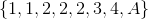and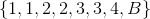are data sets with 2 as their only mode.

Which is the greater quantity?

(a)(b)It cannot be determined which of (a) and (b) is greater

(a) and (b) are equal

(a) is the greater quantity

(b) is the greater quantity

It cannot be determined which of (a) and (b) is greater

Explanation:

The mode of a data set is the element that occurs the most frequently in the set.

In the data set,  if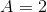, it appears four times, more than any other element. If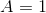, 1 and 2 each appear three times, making the set bimodal, so 2 is not the only mode, but one of two modes. Ifis equal to any other number, 2 appears three times, again, more than any other element. Therefore,can be any number except 1.

In the data set, each of 1, 2, and 3 appears twice. The only value ofthat makes 2 appear the most times, and therefore, the only mode , is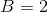.

We therefore know that, but we do not know; we do not know which one is the greater.

### Example Question #15 : How To Find Mode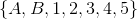is a data set with exactly one mode.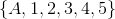is a data set with exactly one mode.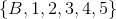is a data set with exactly one mode.

Which is the greater quantity?

(a)(b)It cannot be determined which of (a) and (b) is greater

(b) is the greater quantity

(a) is the greater quantity

(a) and (b) are equal

(a) and (b) are equal

Explanation:

The mode of a set is the element that occurs in the set most frequently. A set without any repeated element is considered to have no mode.

For there to be a mode in the set, it must hold that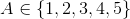; the value ofis the mode of this set. Similarly,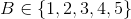..

If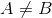, then, in the set, two of the elements in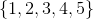appear twice, giving the set two modes. If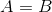, then the value they are equal to appears three times as opposed to the other values, which appear one time each. That gives the set exactly one mode.

### Example Question #16 : How To Find Mode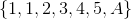and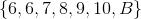are both bimodal sets.

Which is the greater quantity?

(a)(b)(a) and (b) are equal

It cannot be determined which of (a) and (b) is greater

(b) is the greater quantity

(a) is the greater quantity

Consider. If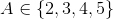, then 1 andeach appear twice, more than any other element; this makes the set bimodal.  If, the set has one mode, 1, since it appears three times, more than any other element. Ifhas any other value, then the set has one mode, 1.  Therefore,. For similar reasons,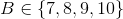. Therefore,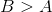.